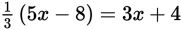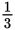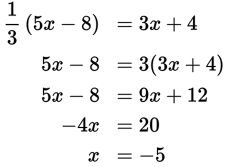# SAT Math Multiple Choice Question 573: Answer and Explanation

### Test Information

Question: 573

3.Which value of x satisfies the equation above?

• A. –5
• B. –3
• C. –1
• D. 1

Explanation:

A

Difficulty: Easy

Category: Heart of Algebra / Linear Equations

Strategic Advice: Choose the best strategy to answer the question. If you distribute the, it creates messy numbers. Instead, clear the fraction by multiplying both sides of the equation by 3. Then use inverse operations to solve for x.